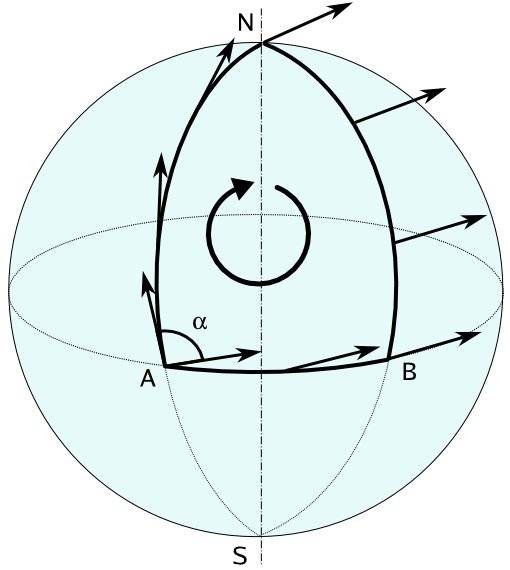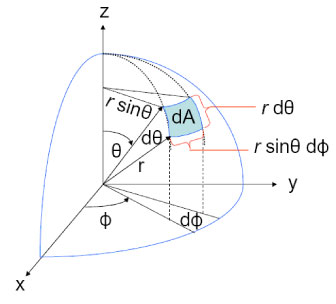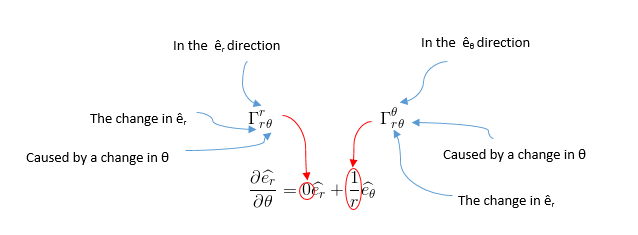So, I’ve decided to bite the bullet and study general relativity. I’ve been postponing it for quite a while, but the idea of my life ending without having studied one of the most profound and fundamental theories of physics was as much disturbing as motivating. I will be posting random stuff as I go and maybe I’ll come back later to edit them, as my understanding of the theory -hopefully- deepens.

I decided to watch the video lectures from Professor Susskind that are publicly available on YouTube. I liked them because Susskind puts an emphasis on the physical aspect of things and less on the formalism of the mathematics. Of course both are required, but for starters I think that it’s best to first build some intuition.

Our today’s goal is to come up with a tool to measure the curvature of space. Generally speaking, a change in the direction of a vector parallel-transported around a closed loop is a way to measure precisely this. Consider the following vector that is parallel-transported across $$A \rightarrow N \rightarrow B \rightarrow A$$.Image taken from here.

And notice how the initial vector and the final vector differ with an angle $$\alpha$$. So, the idea is to compute the commutator of two covariant derivatives. This is as if we first move along the direction $$\mu$$ and then $$\nu$$ vs. first the direction $$\nu$$ and then $$\mu$$. Let us consider the action of this operator on a random vector $$V^\rho$$:

\begin{align*} [\nabla_\mu, \nabla_\nu ] V^\rho &= \nabla_\mu \nabla_\nu V^\rho - \nabla_\nu \nabla_\mu V^\rho \\ &= \nabla_\mu \left[ \partial_\nu V^\rho + \Gamma_{\nu\sigma}^\rho V^\sigma \right] - (\mu \leftrightarrow \nu)\\ &= \partial_\mu \left[ \partial_\nu V^\rho + \Gamma_{\nu\sigma}^\rho V^\sigma \right] - \Gamma_{\mu \nu}^\lambda \left[ \partial_\lambda V^\rho + \Gamma_{\lambda \sigma}^\rho V^ \sigma\right] +\Gamma_{\mu\lambda}^\rho \left[ \partial_\nu V^\lambda + \Gamma_{\nu\sigma}^\lambda V^\sigma\right] - (\mu \leftrightarrow \nu)\\ &= \partial_\mu \partial_\nu V^\rho + \underbrace{\partial_\mu (\Gamma_{\nu\sigma}^\rho) V^\sigma + \Gamma_{\nu\sigma}^\rho \partial_\mu V^\sigma}_{\partial_\mu(\Gamma_{\nu\sigma}^\rho V^\sigma)} -\Gamma_{\mu\nu}^\lambda \partial_\lambda V^\rho - \Gamma_{\mu\nu}^\lambda \Gamma_{\lambda \sigma}^\rho V^\sigma + \Gamma_{\mu\lambda}^\rho \partial_\nu V^\lambda + \Gamma_{\mu\lambda}^\rho \Gamma_{\nu\sigma}^\lambda V^\sigma\\ &-\partial_\nu\partial_\mu V^\rho - \underbrace{\partial_\nu (\Gamma_{\mu\sigma}^\rho) V^\sigma - \Gamma_{\mu\sigma}^\rho \partial_\nu V^\sigma}_{\partial_\nu(\Gamma_{\mu\sigma}^\rho V^\sigma)} +\Gamma_{\nu\mu}^\lambda \partial_\lambda V^\rho + \Gamma_{\nu\mu}^\lambda \Gamma_{\lambda \sigma}^\rho V^\sigma - \Gamma_{\nu\lambda}^\rho \partial_\mu V^\lambda - \Gamma_{\nu\lambda}^\rho \Gamma_{\mu\sigma}^\lambda V^\sigma\\ &= \underbrace{\left[ \partial_\mu \Gamma_{\nu\sigma}^\rho - \partial_\nu \Gamma_{\mu\sigma}^\rho + \Gamma_{\mu\lambda}^\rho \Gamma_{\nu\sigma}^\lambda - \Gamma_{\nu\lambda}^\rho \Gamma_{\mu\sigma}^\lambda \right]}_{R_{\sigma\mu\nu}^\rho} V^\sigma\\ &= R_{\sigma\mu\nu}^\rho V^\sigma \end{align*}

The directions $$\mu$$ and $$\nu$$ are our two transport directions, whereas $$\sigma$$ is out initial direction. The tensor $$R_{\sigma\mu\nu}^\rho$$ tells us the difference of the vectors obtained by transporting $$\partial\sigma$$ first along $$\nu$$ and then along $$\mu$$ directions vs. the same vector obtained by first transporting along $$\mu$$ and then $$\nu$$. The index $$\rho$$ is the $$\rho$$-th component.

Some useful tips for the above calculation:

• The covariant derivative of a type $$(r,s)$$ tensor field along $$\mu$$ is given by the expression: \begin{align} {(\nabla_\mu T)^{a_1 \ldots a_r}}_{b_1 \ldots b_s} = {} &\frac{\partial}{\partial x^\mu}{T^{a_1 \ldots a_r}}_{b_1 \ldots b_s} \\ &+ \,{\Gamma ^{a_1}}_{\lambda\mu} {T^{\lambda a_2 \ldots a_r}}_{b_1 \ldots b_s} + \cdots + {\Gamma^{a_r}}_{\lambda\mu} {T^{a_1 \ldots a_{r-1}\lambda}}_{b_1 \ldots b_s} \\ &-\,{\Gamma^\lambda}_{b_1 \mu} {T^{a_1 \ldots a_r}}_{\lambda b_2 \ldots b_s} - \cdots - {\Gamma^\lambda}_{b_s \mu} {T^{a_1 \ldots a_r}}_{b_1 \ldots b_{s-1} \lambda}. \end{align}

Meaning that you take the ordinary partial derivative of the tensor and then add $$+{\Gamma^{a_i}}_{\lambda \mu}$$ for every upper index $$a_i$$ and $$-{\Gamma^\lambda}_{b_i \mu}$$ for every lower index $$b_i$$.

• There are generalized Riemannian geometries that have torsion, in which the symmetry $$\Gamma_{\mu\nu}^\lambda = \Gamma_{\nu \mu}^\lambda$$ does not hold. Those geometries are not widely used in ordinary gravitational theory. The geometry of general relativity is the Minkowski-Einstein geometry which is an extension of Riemannian geometry with a non-positive definite metric, but it doesn’t involve torsion.

• Quantities that have different summation indices, but otherwise have the same symbols, are equal and cancel each other. For instance, $$\Gamma_{\mu\lambda}^\rho \partial_\nu V^\lambda$$ is equal to $$\Gamma_{\mu\sigma}^\rho \partial_\nu V^\sigma$$, because indices $$\lambda$$ and $$\sigma$$ are used just as dummy indices for the summation.

### Example

Let us calculate the curvature of the surface of a sphere. To do that we need the Christoffel symbols $$\Gamma_{\mu\nu}^\lambda$$ and since these symbols are expressed in terms of the partial derivatives of the metric tensor, we need to calculate the metric tensor $$g_{\mu\nu}$$.

#### Calculation of metric tensor $$g_{\mu\nu}$$

The following image illustrates the calculation of infinitestimal length $$\mathrm{d}S^2$$ on the surface of a sphere.$\mathrm{d}S^2 = \left(R \mathrm{d}\theta \right)^2 + (R\sin\theta \mathrm{d}\phi)^2 = R^2 \left( \mathrm{d}\theta^2 + \sin^2\theta \mathrm{d}\phi^2 \right)$

But recall that:

\begin{align*} \mathrm{d}S^2 &= g_{\mu\nu} \mathop{\mathrm{d}x^\mu}\mathop{\mathrm{d}x^\nu } = \sum_{\mu=1}^2 \sum_{\nu=1}^2 g_{\mu\nu} \mathop{\mathrm{d}x^\mu}\mathop{\mathrm{d}x^\nu }\\ &= g_{11} \mathrm{d}x^1 \mathrm{d}x^1 + \underbrace{g_{12} \mathrm{d}x^1 \mathrm{d}x^2 + g_{21} \mathrm{d}x^2 \mathrm{d}x^1}_{\text{Equal due to symmetry}} + g_{22} \mathrm{d}x^2 \mathrm{d}x^2\\ &= g_{11} (\mathrm{d}x^1)^2 + 2g_{12} \mathrm{d}x^1 \mathrm{d}x^2 + g_{22} (\mathrm{d}x^2)^2 \end{align*}

Let us use $$x^1 = \theta$$ and $$x^2 = \phi$$, then:

\begin{align*} dS^2 &= g_{\theta\theta} (\mathrm{d}\theta)^2 + 2g_{\theta\phi} \mathop{\mathrm{d}\theta} \mathop{\mathrm{d}\phi} &+ &g_{\phi\phi} (\mathrm{d}\phi)^2\\ &=R^2 \mathrm{d}\theta^2 &+ &R\sin^2\theta \mathrm{d}\phi^2 \end{align*}

Therefore:

$g_{\theta\theta} = R^2, g_{\theta\phi} = 0, g_{\phi\phi} = R\sin^2\theta$

And in matrix notation: $$\begin{pmatrix} g_{\theta \theta} & g_{\theta \phi} \\ g_{\phi \theta} & g_{\phi \phi} \end{pmatrix}= \begin{pmatrix} R^2 & 0 \\ 0 & R^2\sin^2\theta \end{pmatrix}$$

#### Calculation of the Christoffel symbols

Let us recall that the Christoffel symbol of the form $$\Gamma_{\mu\nu}^\lambda$$ is given by the following formula:

$\Gamma_{\mu\nu}^\lambda = \frac{1}{2}g^{\lambda\sigma} \left( \frac{\partial g_{\sigma\nu}}{\partial x^\mu} + \frac{\partial g_{\sigma\mu}}{\partial x^\nu} - \frac{\partial g_{\mu\nu}}{\partial x^\sigma}\right)= \frac{1}{2}g^{\lambda\sigma} \left( \partial_\mu g_{\sigma\nu} + \partial_\nu g_{\sigma\mu} - \partial_\sigma g_{\mu\nu}\right)$

At this point I’m going to cheat. Here is a function in Mathematica that calculates the $$\Gamma_{\mu\nu}^\lambda$$:

Or a little prettier:

$\Gamma_{\mu\nu}^\theta = \left( \begin{array}{cc} 0 & 0 \\ 0 & -\sin \theta \cos \theta \\ \end{array} \right)\qquad \Gamma_{\mu\nu}^\phi = \left( \begin{array}{cc} 0 & \cot \theta \\ \cot \theta & 0 \\ \end{array} \right)$

Let us remind ourselves of what the Christoffel symbol $$\Gamma_{\mu\nu}^\lambda$$ means:Image taken from here.

Now we are onto the calculation of the Riemann curvature tensor:

Let us calculate the component $$R_{\phi\theta\phi}^\theta$$ for example.

Or, in pretty format:

$R = \left( \begin{array}{cc} \left( \begin{array}{cc} 0 & 0 \\ 0 & 0 \\ \end{array} \right) & \left( \begin{array}{cc} 0 & \sin^2 \theta \\ -\sin^2 \theta & 0 \\ \end{array} \right) \\ \left( \begin{array}{cc} 0 & -1 \\ 1 & 0 \\ \end{array} \right) & \left( \begin{array}{cc} 0 & 0 \\ 0 & 0 \\ \end{array} \right) \\ \end{array} \right)$

Therefore, $$R_{\phi\theta\phi}^\theta = \sin^2\theta$$. Sometimes it’s more convenient to write the fully covariant version of the Riemann tensor (that is the tensor with all indices lowered), e.g.

\begin{align*} R_{\rho\sigma\mu\nu} &= g_{\rho \xi} R_{\sigma\mu\nu}^\xi \Rightarrow\\ R_{\theta\phi\theta\phi} &= g_{\theta \xi} R_{\phi\theta\phi}^\xi = \sum_{\xi=1}^2 g_{\theta \xi} R_{\phi\theta\phi}^\xi = g_{\theta \theta} R_{\phi\theta\phi}^\theta + g_{\theta \phi} R_{\phi\theta\phi}^\phi = R^2 \sin^2\theta \end{align*}

since $$g_{\theta\theta} = R^2$$ and $$g_{\theta\phi} = 0$$.